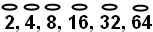#### You may also like### Tweedle Dum and Tweedle Dee

Two brothers were left some money, amounting to an exact number of pounds, to divide between them. DEE undertook the division. "But your heap is larger than mine!" cried DUM...### Sum Equals Product

The sum of the numbers 4 and 1 [1/3] is the same as the product of 4 and 1 [1/3]; that is to say 4 + 1 [1/3] = 4 � 1 [1/3]. What other numbers have the sum equal to the product and can this be so for any whole numbers?### Special Sums and Products

Find some examples of pairs of numbers such that their sum is a factor of their product. eg. 4 + 12 = 16 and 4 × 12 = 48 and 16 is a factor of 48.

# The Greedy Algorithm

### Why do this problem?

This problem follows on from Keep It Simple and Egyptian Fractions
These three problems together offer students an opportunity to engage with some mathematical ideas in depth and not just with the rather mechanical process of adding and subtracting fractions.

This problem in particular requires students to compare fractions and may deepen their understanding of their relative sizes.

### Possible approach

Students should already have worked with fractions of the form $\frac{1}{n}$, $\frac{2}{n}$ and possibly $\frac{3}{n}$ and $\frac{4}{n}$ in Keep It Simple and Egyptian Fractions .

To give students a 'feel' for the difficulty of expressing fractions as the Egyptians did, ask students to work in pairs for a short time finding an Egyptian sum for any fraction of their choice. Note, all the unit fractions in the summation must be unique. Any that they can't do can be written on the board for others to attempt.

Draw the group together and share strategies. Compare their efficiency. Do they always give the same result?

If you start with $\frac{4}{5}$, for example, and apply students' different strategies, you may end up with different outcomes:

$\frac{4}{5} = \frac{1}{2} + \frac{1}{4} + \frac{1}{20}$ or

$\frac{4}{5} = \frac{1}{2} + \frac{1}{5} + \frac{1}{10}$ or
$\frac{4}{5} = \frac{1}{3} + \frac{1}{5} + \frac{1}{6} + \frac{1}{15} + \frac{1}{30}$
....
If no-one has suggested it, introduce the Greedy Algorithm and check to see if anyone has used it already.

Ask students to choose fractions of their own and apply the Greedy Algorithm. Does it always work?

Can they find a convincing explanation?

### Key Questions

Is our fraction larger or smaller than $\frac{1}{2}$? How do we know?

Is our fraction larger or smaller than $\frac{1}{3}$? How do we know?
Is our fraction larger or smaller than $\frac{1}{4}$? How do we know?

....

### Possible support

Students who need support in comparing the size of fractions might have to do some preliminary work on equivalent fractions.

Alternatively, they could convert them to decimal equivalents using a calculator.

### Possible extension

Students could try to prove why Fibonnacci's Greedy Algorithm always terminates (the numerators always decrease and must therefore reach one).

Does the Greedy Algorithm always result in the sum with the fewest possible terms?

Can anyone find a counter example?

The Eye of Horus: often it was good enough to use only the fractionsthat represent $\frac{1}{2}$, $\frac{1}{4}$, $\frac{1}{8}$.... to get a fraction that is close enough to any specific fraction. Suggest that students pick some fractions and convert them to this form of Egyptian fraction.
How close does this method get to the target fraction?
Students might like to research how these particular fractions were written down in pictorial form.